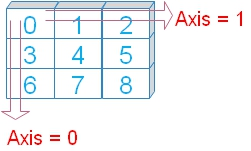# Pandas DataFrame sum()

We can sum number of in rows or columns by using sum().

``````import pandas as pd
my_dict={'NAME':['Ravi','Raju','Alex','Ron','King','Jack'],
'ID':[1,2,3,4,5,6],
'MATH':[80,40,70,70,70,30],
'ENGLISH':[80,70,40,50,60,30]}
my_data = pd.DataFrame(data=my_dict)
print(my_data.sum())``````
Output
``````NAME       RaviRajuAlexRonKingJack
ID                              21
MATH                           360
ENGLISH                        330``````

## Using axisWe will use option axis=0 ( default ) by adding to above code.

( The last line is only changed )
``print(my_data.sum(axis=1))``
Output is here
``````0    161
1    112
2    113
3    124
4    135
5     66``````

## level option

Sum of two column values.
``````import pandas as pd
my_dict={
'name':['Alex','King','Ravi','Raju','John'],
'mark':[7,8,5,6,3],
'math':[70,80,50,60,30]
}
df = pd.DataFrame(data=my_dict) # create DataFrame
df.set_index('name',inplace=True) # added index to Name column
print(df[['math','mark']].sum())``````
Sum of column values ( of all rows ) Output
``````math    290
mark     29
dtype: int64``````
Using Axis ( In above code default value for axis = 0 ), We can use axis=1 to sum values of columns in each row.
``print(df[['math','mark']].sum(axis=1))``
Output
``````name
Alex    77
King    88
Ravi    55
Raju    66
John    33
dtype: int64``````
For MultiIndex (hierarchical) axis we can specify the level.
``````import pandas as pd
my_dict=pd.MultiIndex.from_arrays(
[[1,2,3,4,5,6],
[80,40,70,70,70,30],
[80,70,40,50,60,30]],
names=['id','math','eng'])
my_data = pd.Series([4, 2, 0, 8,3,4], name='marks', index=my_dict)
print(my_data.sum(level='math'))``````
Output
``````math
80     4
40     2
70    11
30     4``````

## Handling NA data

We will use skipna=True to ignore the null or NA data. Let us check what happens if it is set to True ( skipna=True )
``````import numpy as np
import pandas as pd
my_dict={'NAME':['Ravi','Raju','Alex','Ron','King','Jack'],
'ID':[1,2,3,4,5,6],
'MATH':[80,40,70,70,70,30],
'ENGLISH':[80,70,np.nan,50,60,30]}
my_data = pd.DataFrame(data=my_dict)
print(my_data.sum(skipna=False))``````
Output
``````NAME       RaviRajuAlexRonKingJack
ID                              21
MATH                           360
ENGLISH                        NaN``````
Check the sum of ENGLISH it is returned as NAN, the value will change to 290 if we set skipna=True

## numeric_only

Default value is None, we can set it to True ( numeric_only=True ) to include only float, int, boolean columns. We can included all by setting it to False ( numeric_only=False ) . Let us see the outputs .
``print(my_data.sum(numeric_only=False))``
Output is here
``````NAME       RaviRajuAlexRonKingJack
ID                              21
MATH                           360
ENGLISH                      330.5``````
Now let us change the option numeric_only to True ( numeric_only=True ).
``print(my_data.sum(numeric_only=True))``
Output
``````ID          21.0
MATH       360.0
ENGLISH    330.5``````

## min_count

Default value is 0, it accepts int. It is required number of valid values to perform.

Subscribe to our YouTube Channel here

## Subscribe

* indicates required
Subscribe to plus2netplus2net.com